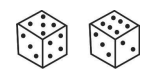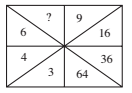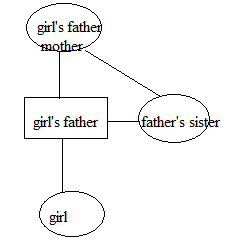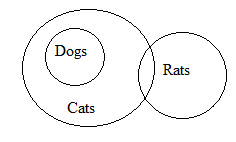# SSC CGL 2018 Practice Test Papers | Reasoning Ability (Day-23)

Dear Aspirants, Here we have given the Important SSC CGL Exam 2018 Practice Test Papers. Candidates those who are preparing for SSC CGL 2018 can practice these questions to get more confidence to Crack SSC CGL 2018 Examination.

[WpProQuiz 3008]

Click “Start Quiz” to attend these Questions and view Explanation

1) A girl pointing to a man and said” He is the only brother of daughter of my father’s mother. How is the man related to that girl?

a)Uncle

b)Grandfather

c)Father

d) Son

2) Statement:

All dogs are cats. Some cats are rats.

Conclusion:

1. All rats are cats.
2. Some rats are cats.

a) Only I is follows

b) Only II is follows

c) Both I and II Follow

d) Neither I nor II follows

3) AB:DH::GN:??

a) MZ

b) NX

c) SP

d) RP

4) Select the related number from the given alternatives

25:29 :: 35 : ??

a) 39

b) 34

c) 31

d) 18

5) Complete the series given below.

3, 5, 13, 43, 177, ??

a) 900

b) 450

c) 689

d) 891

6) If ‘x’ means ‘addition’, ‘-‘ means ‘division’, ‘/’ means ‘subtraction’ and ‘+’ means ‘multiplication’, then what will be the value of the expression ’10+6-10/2×25-5′?

a) 10

b) 9

c) 3

d) 12

7) Two positions of a dice are shown below. If 1 is at the bottom, which number will be on top?a) 4

b) 3

c) 8

d) 5

8) In a certain code language STEM is written as 5% * K and COME is written as \$7 K*. How is COST written in that code?

(a) 5% K5

(b) 5 7K5

(c) \$75%

(d) 5\$%5

9) In the following question, which one set of letters when sequentially placed at the gaps in the given letter series shall complete it? d _ ba_c_a_cb_

a) cdbda

c) bdacd

d) abdca

10) Which of the following numbers should be in blank space?a) 3

b) 4

c) 8

d) 6A(1)       B(2); 1×2=2

D(4)       H(8); 4×2=8

G(7)       N(14); 7×2=14

M(13)    Z(26);13×2=26

22+52=29

32+52=34

3×1+2 = 5

5×2+3 = 13

13×3+4 = 43

43×4+5 = 177

177×5+6 = 891

10×6/10-2+25/5=9

2, 4, 5 and 6 are adjacent faces of 3. Therefore, if number 1 is at the bottom then 3 will be on the top.

Here, C=> \$, O=>7, E => *, M=> K, S=> 5, T=> % Therefore, COST=> \$75%

dcba/ dcba/ dcba